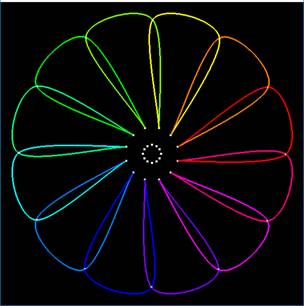## Geometric Modeling

This page was last updated: September 12, 2022### Introduction:

The goal of this project is to create an animated scene of Catmull-Rom curves.

1. It must be in 3D (i.e., Z cannot be constant).
2. You must have at least 1 stationary curve.
3. You must have at least 5 animating curves.
4. The curves must somehow be "different" from each other. That could mean rotated, scaled, different points, etc. Just translated is not enough.

### Requirements:

1. Create a scene of at least 6 3D Catmull-Rom curves.
2. What the scene looks like is up to you. 10 of the 100 project points are given if you deliberately make it look like something at least somewhat recognizeable. You lose those 10 points if it looks like you created this scene by accident.
3. How you color the scene is up to you.
4. How you animate the scene is up to you.
5. How many points you use to draw each curve is up to you, but, use enough points in the GL_LINE_STRIP to make the curves look smooth. Use a Δt = 1./(the number of points-1).
6. Animate each curve by changing the position of one (or more) points per curve based on the Time variable you have been creating in the last couple of projects.
7. Be able to turn the control points on and off.
8. Be able to turn the control lines on and off. (A "control line" is a line strip connecting the curve's first endpoint point to the control points in order, to the last endpoint.)
9. Enable the following keys:
 'f' Freeze/un-freeze the animation
(This isn't a requirement, but it always helps to debug animations.)

### Turn-in:

Use the Teach system to turn in your:

1. Your PDF report, describing what you did and where we can find your video. Be sure your video makes it obvious that you are doing this in 3D!
2. Your .cpp file

### Possible Way to Organize the Data

```
struct Point
{
float x0, y0, z0;       // initial coordinates
float x,  y,  z;        // animated coordinates
};

struct Curve
{
float r, g, b;
Point p0, p1, p2, p3;
};

Curve Curves[NUMCURVES];		// if you are creating a pattern of curves
Curve Stem;				// if you are not
```

### Reminder: The Cubic Catmull-Rom Equation

P = 0.5 * [ 2.*P1 + t*(-P0+P2) + t2*(2.*P0-5.*P1+4.*P2-P3) + t3*(-P0+3.*P1-3.*P2+P3) ]

0. ≤ t ≤ 1.

### Rotating a Point by an Angle about the X Axis Around a Center

```
void
RotateX( Point *p, float deg, float xc, float yc, float zc )
{
float rad = deg * (M_PI/180.f);         // radians
float x = p->x0 - xc;
float y = p->y0 - yc;
float z = p->z0 - zc;

float xp = x;

p->x = xp + xc;
p->y = yp + yc;
p->z = zp + zc;
}
```

### Rotating a Point by an Angle about the Y Axis Around a Center

```
void
RotateY( Point *p, float deg, float xc, float yc, float zc )
{
float rad = deg * (M_PI/180.f);         // radians
float x = p->x0 - xc;
float y = p->y0 - yc;
float z = p->z0 - zc;

float yp =  y;

p->x = xp + xc;
p->y = yp + yc;
p->z = zp + zc;
}
```

### Rotating a Point by an Angle about the Z Axis Around a Center

```
void
RotateZ( Point *p, float deg, float xc, float yc, float zc )
{
float rad = deg * (M_PI/180.f);         // radians
float x = p->x0 - xc;
float y = p->y0 - yc;
float z = p->z0 - zc;

float zp = z;

p->x = xp + xc;
p->y = yp + yc;
p->z = zp + zc;
}
```

### Drawing a Catmull-Rom Curve:

```
glLineWidth( 3. );
glColor3f( r, g, b );
glBegin( GL_LINE_STRIP );
for( int it = 0; it <= NUMPOINTS; it++ )
{
float t = (float)it / (float)NUMPOINTS;
float x = . . .
float y = . . .
float z = . . .
glVertex3f( x, y, z );
}
glEnd( );
glLineWidth( 1. );
```

### How Did Jane Graphics Get That Nice Color Progression?

She used the hue-saturation-value color scale and the HsvRgb( ) function that is included in your sample code. As long as you are using angles to locate points, you can use that same angle to assign a hue and then turn it into an RGB. See the HSV slide of the Getting Started notes.